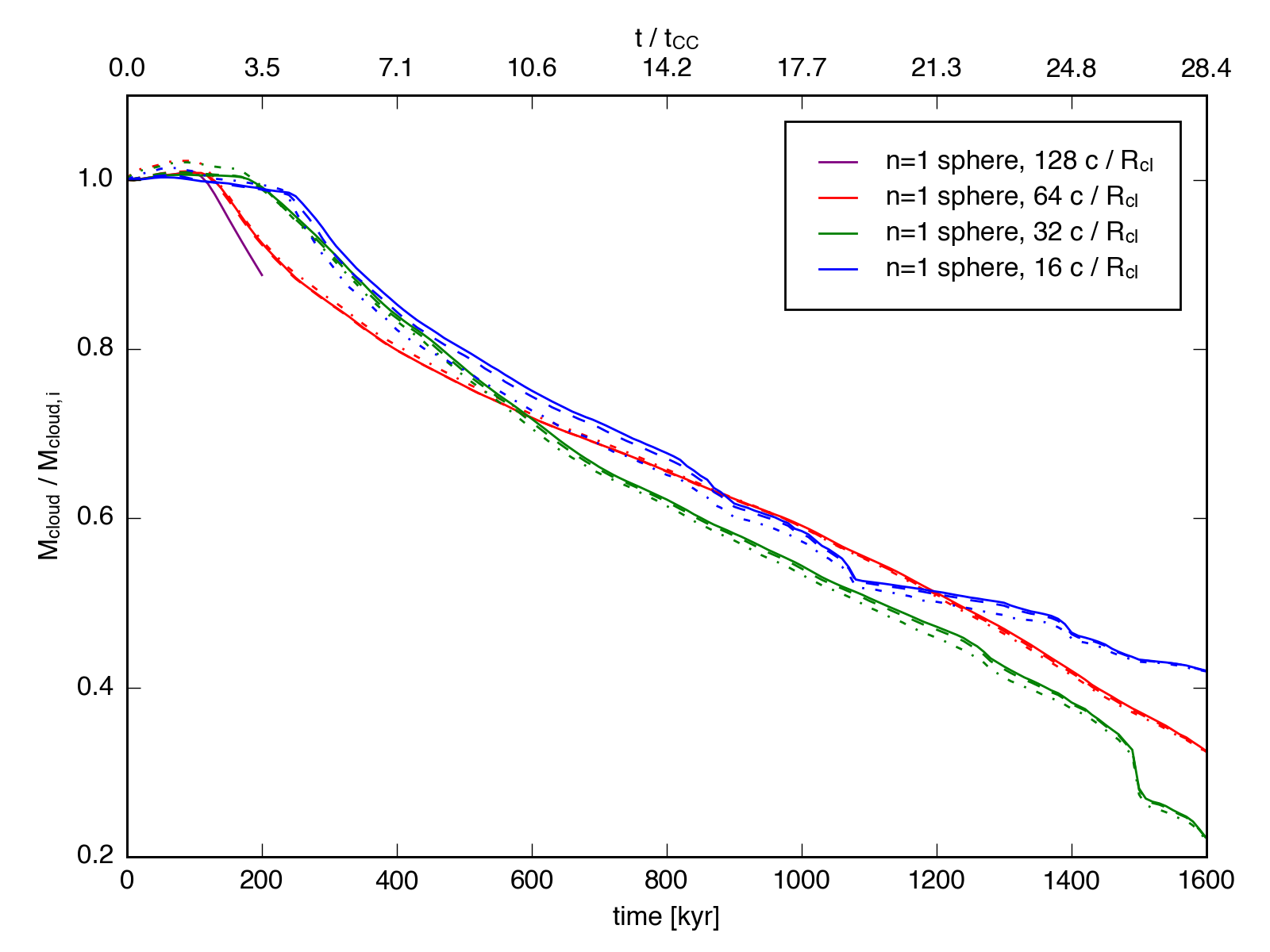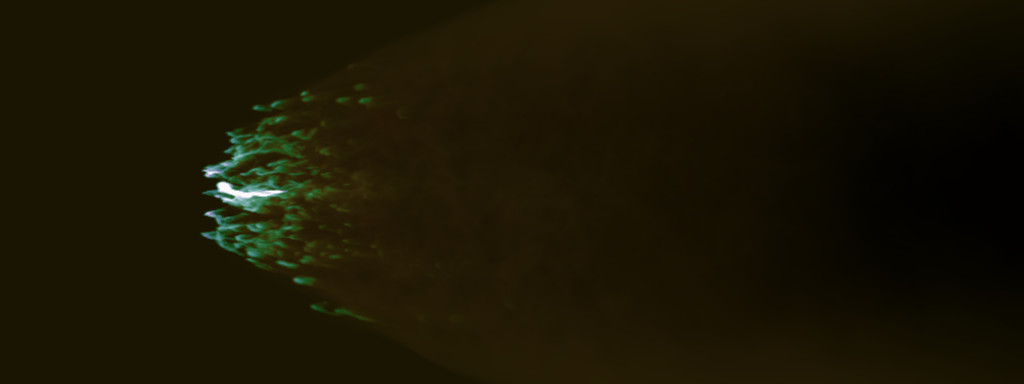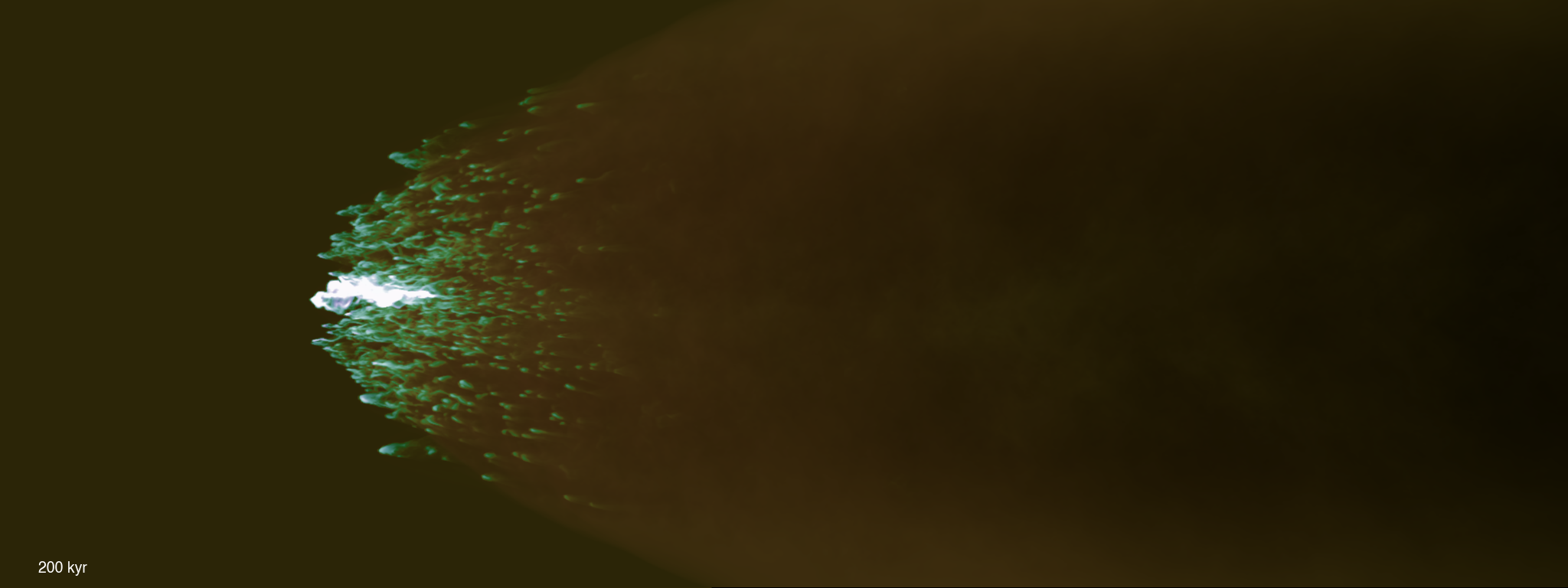Convergence

April 21, 2016

The plot below shows the evolution of the mass fraction for the $n_h = 1$ sphere-wind simulation at four different resolutions. The lowest-resolution sim had 16 cells / $R_\mathrm{cl}$, followed by 32 cells / $R_\mathrm{cl}$, 64 cells / $R_\mathrm{cl}$, and 128 cells / $R_\mathrm{cl}$. All simulations were run on a grid with physical size $160\times40\times40$ pc, except the highest, which was run in an $80\times30\times30$ pc box, and only until $t = 200$ kyr. Lines show the mass fraction calculated as the fraction of material above 1/20th the intitial median density (solid), 1/10th the initial median density (dashed), and 1/3rd the intial median density (dash-dot).In addition, below are snapshots of the clouds at 200 kyr: